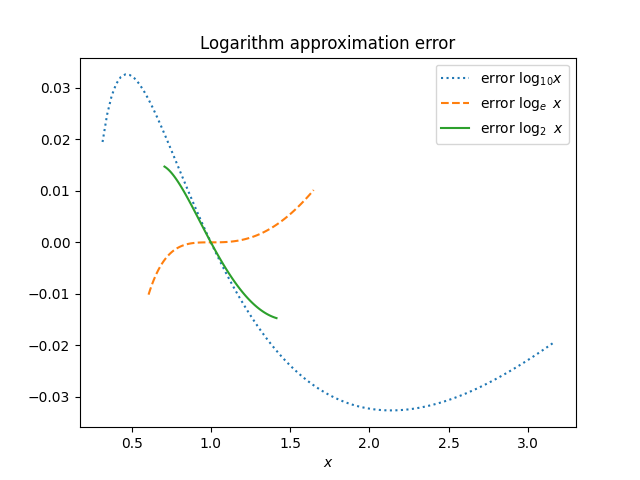# Curiously simple approximations

As I’ve written about here and elsewhere, the following simple approximations are fairly accurate.

log10 x ≈ (x-1)/(x+1)

loge x ≈ 2 (x – 1)/(x + 1)

log2 x ≈ 3(x – 1)/(x + 1)

It’s a little surprising that each is as accurate as it is, but it’s also surprising that the approximations for loge and loge are small integer multiples of the approximation for log10.

Logarithms in all bases are proportional: If b and β are two bases, then for all x,

logβ(x) = logb(x) / logb(β).

So it’s not surprising that the approximations above are proportional. But the proportionality constants are not what the equation above would predict.

There are a couple things going on. First, these approximations are intended for rough mental calculations, so round numbers are desirable. Second, we want to minimize the error over some range. The approximation for logb(x) needs to work over the interval from 1/√b to √b. The approximations don’t work outside this range, but they don’t need to since you can always reduce the problem to computing logs in this interval. These two goals work together.

We could make the log10 x approximation more accurate near 1 if we multiplied it by 0.9. That would make the approximation a little harder to use, but it wouldn’t improve the accuracy over the interval 1/√10 to √10. The constant 1 works just as well as 0.9, and it’s easier to multiply by 1.

Here’s a plot of the approximation errors. Notice that different bases are plotted over different ranges since the log for each base b needs to work from 1/√b to √b.